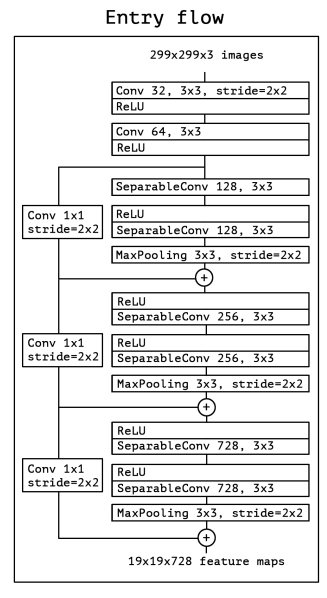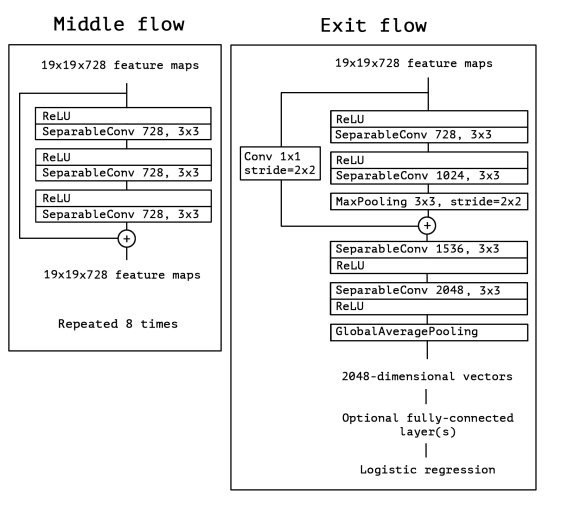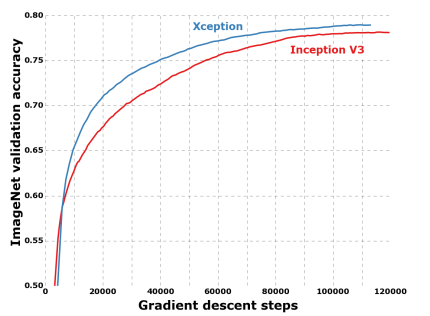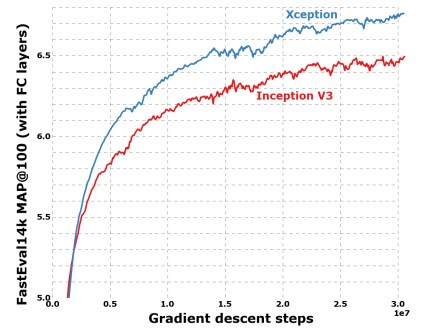### Xception: Implementing from scratch using Tensorflow

#### Even better than Inception

Convolutional Neural Networks (CNN) have come a long way, from the LeNet-style, AlexNet, VGG models, which used simple stacks of convolutional layers for feature extraction and max-pooling layers for spatial sub-sampling, stacked one after the other, to Inception and ResNet networks which use skip connections and multiple convolutional and max-pooling blocks in each layer. Since its introduction, one of the best networks in computer vision has been the Inception network. The Inception model uses a stack of modules, each module containing a bunch of feature extractors, which allow them to learn richer representations with fewer parameters.

Xception paper — https://arxiv.org/abs/1610.02357

As we see in figure 1, the Xception module has 3 main parts. The Entry flow, the Middle flow (which is repeated 8 times), and the Exit flow.Figure 2. Entry flow of the Xception architecture (Source: Image from the original paper)

The entry flow has two blocks of convolutional layer followed by a ReLU activation. The diagram also mentions in detail the number of filters, the filter size (kernel size), and the strides.

There are also various Separable convolutional layers. There are also Max Pooling layers. When the strides are different than one, the strides are also mentioned. There are also Skip connections, where we use ‘ADD’ to merge the two tensors. It also shows the shape of the input tensor in each flow. For example, we begin with an image size of 299x299x3, and after the entry flow, we get an image size of 19x19x728.Figure 3. Middle and Exit flow of Xception architecture (Source: Image from the original paper)

Similarly, for the Middle flow and the Exit flow, this diagram clearly explains the image size, the various layers, the number of filters, the shape of filters, the type of pooling, the number of repetitions, and the option of adding a fully connected layer in the end.

Also, all Convolutional and Separable Convolutional layers are followed by batch normalization.

#### Separable Convolutional Layer

Separable convolutions consist of first performing a depthwise spatial convolution (which acts on each input channel separately) followed by a pointwise convolution which mixes the resulting output channels.- From Keras Documentation

Let's assume that we have an input tensor of size (K, K,3). K is the spatial dimension and 3 is the number of feature maps/channels. As we see from the above Keras documentation, first we need to implement depthwise spatial convolution on each input channel separately. So we use K, K,1 — the first channel of the image/tensor. Suppose we use a filter of size 3x3x1. And this filter is applied across all three channels of the input tensor. As there are 3 channels, so the dimension we get is 3x3x1x3. This is shown in the Depthwise convolution part of Figure 4.

After this, all the 3 outputs are taken together, and we obtain a tensor of size (L, L,3). The dimensions of L can be the same as K or can be different, depending on the strides and padding used in the previous convolutions.

Then the Pointwise convolution is applied. The filter is of size 1x1x3 (3 channels). And the number of filters can be any number of filters we want. Let’s say we use 64 filters. So the total dimension comes to 1x1x3x64. Finally, we obtain an output tensor of size LxLx64. this is shown in the Pointwise convolution part of Figure 4.

Why is separable convolution better than normal convolution?

If we were to use a normal convolution on the input tensor, and we use a filter/kernel size of 3x3x3 (kernel size — (3,3) and 3 feature maps). And the total number of filters we want is 64. So a total of 3x3x3x64.

Instead, in separable convolution, we first use 3x3x1x3 in depthwise convolution and 1x1x3x64 in pointwise convolution.

The difference lies in the dimensionality of the filters.

Traditional Convolutional layer = 3x3x3x64 = 1,728

Separable Convolutional layer = (3x3x1x3)+(1x1x3x64) = 27+192 = 219

As we see, separable convolution layers are way more advantageous than traditional convolutional layers, both in terms of computation cost as well as memory. The main difference is that in the normal convolution, we are transforming the image multiple times. And every transformation uses up 3x3x3x64 = 1,728 multiplications. In the separable convolution, we only transform the image once — in the depthwise convolution. Then, we take the transformed image and simply elongate it to 64 channels. Without having to transform the image over and over again, we can save up on computational power.Figure 5. Xception performance vs Inception on ImageNet (Source: Image from the original paper)Figure 6. Xception performance vs Inception on JFT dataset (Source: Image from the original paper)

Algorithm:

1. Import all the necessary layers
2. Write all the necessary functions for:

a. Conv-BatchNorm block

b. SeparableConv- BatchNorm block

3. Write one function for each one of the 3 flows — Entry, Middle, and Exit

4. Use these functions to build the complete model

### Creating Xception using Tensorflow

`#import necessary librariesimport tensorflow as tffrom tensorflow.keras.layers import Input,Dense,Conv2D,Addfrom tensorflow.keras.layers import SeparableConv2D,ReLUfrom tensorflow.keras.layers import BatchNormalization,MaxPool2Dfrom tensorflow.keras.layers import GlobalAvgPool2Dfrom tensorflow.keras import Model`

Creating the Conv-BatchNorm block:

`# creating the Conv-Batch Norm blockdef conv_bn(x, filters, kernel_size, strides=1):        x = Conv2D(filters=filters,                kernel_size = kernel_size,                strides=strides,                padding = 'same',                use_bias = False)(x)    x = BatchNormalization()(x)`
`return x`

The Conv-Batch Norm block takes as inputs, a tensor — x, number of filters — filters, kernel size of the convolutional layer — kernel_size, strides of convolutional layer — strides. Then we apply a convolution layer to x and then apply Batch Normalization. We add use_bias = False, so that the number of parameters of the final model, will be the same as the number of parameters of the original paper.

Creating the SeparableConv- BatchNorm block:

`# creating separableConv-Batch Norm blockdef sep_bn(x, filters, kernel_size, strides=1):        x = SeparableConv2D(filters=filters,                         kernel_size = kernel_size,                         strides=strides,                         padding = 'same',                         use_bias = False)(x)    x = BatchNormalization()(x)`
`return x`

Similar structure as the Conv-Batch Norm block, except we use SeparableConv2D instead of Conv2D.

Functions for Entry, Middle, and Exit flow:

`# entry flowdef entry_flow(x):        x = conv_bn(x, filters =32, kernel_size =3, strides=2)    x = ReLU()(x)    x = conv_bn(x, filters =64, kernel_size =3, strides=1)    tensor = ReLU()(x)        x = sep_bn(tensor, filters = 128, kernel_size =3)    x = ReLU()(x)    x = sep_bn(x, filters = 128, kernel_size =3)    x = MaxPool2D(pool_size=3, strides=2, padding = 'same')(x)        tensor = conv_bn(tensor, filters=128, kernel_size = 1,strides=2)    x = Add()([tensor,x])        x = ReLU()(x)    x = sep_bn(x, filters =256, kernel_size=3)    x = ReLU()(x)    x = sep_bn(x, filters =256, kernel_size=3)    x = MaxPool2D(pool_size=3, strides=2, padding = 'same')(x)        tensor = conv_bn(tensor, filters=256, kernel_size = 1,strides=2)    x = Add()([tensor,x])        x = ReLU()(x)    x = sep_bn(x, filters =728, kernel_size=3)    x = ReLU()(x)    x = sep_bn(x, filters =728, kernel_size=3)    x = MaxPool2D(pool_size=3, strides=2, padding = 'same')(x)        tensor = conv_bn(tensor, filters=728, kernel_size = 1,strides=2)    x = Add()([tensor,x])`
`return x`

Here we just follow Figure 2. It begins with two Conv layers with 32 and 64 filters respectively. Each followed by a ReLU activation.

Then there is a skip connection, which is done by using Add.

Inside each of the skip connection blocks, there are two separable Conv layers followed by MaxPooling. The skip connections itself have a Conv layer of 1x1 with strides 2.

`# middle flowdef middle_flow(tensor):        for _ in range(8):        x = ReLU()(tensor)        x = sep_bn(x, filters = 728, kernel_size = 3)        x = ReLU()(x)        x = sep_bn(x, filters = 728, kernel_size = 3)        x = ReLU()(x)        x = sep_bn(x, filters = 728, kernel_size = 3)        x = ReLU()(x)        tensor = Add()([tensor,x])            return tensor`

The middle flow follows the steps as shown in figure 7.

`# exit flowdef exit_flow(tensor):        x = ReLU()(tensor)    x = sep_bn(x, filters = 728,  kernel_size=3)    x = ReLU()(x)    x = sep_bn(x, filters = 1024,  kernel_size=3)    x = MaxPool2D(pool_size = 3, strides = 2, padding ='same')(x)        tensor = conv_bn(tensor, filters =1024, kernel_size=1, strides =2)    x = Add()([tensor,x])        x = sep_bn(x, filters = 1536,  kernel_size=3)    x = ReLU()(x)    x = sep_bn(x, filters = 2048,  kernel_size=3)    x = GlobalAvgPool2D()(x)        x = Dense (units = 1000, activation = 'softmax')(x)        return x`

The exit flow follows the steps as shown in figure 8.

Creating the Xception Model:

`# model codeinput = Input(shape = (299,299,3))x = entry_flow(input)x = middle_flow(x)output = exit_flow(x)model = Model (inputs=input, outputs=output)model.summary()`

Output snippet:

`from tensorflow.python.keras.utils.vis_utils import model_to_dotfrom IPython.display import SVGimport pydotimport graphvizSVG(model_to_dot(model, show_shapes=True, show_layer_names=True, rankdir='TB',expand_nested=False, dpi=60, subgraph=False).create(prog='dot',format='svg'))`

Output snippet:

`import numpy as np import tensorflow.keras.backend as K `
`np.sum([K.count_params(p) for p in model.trainable_weights])`

Output: 22855952

The above code displays the number of trainable parameters.

Entire code to create Xception model from scratch using Tensorflow:

`#import necessary librariesimport tensorflow as tffrom tensorflow.keras.layers import Input,Dense,Conv2D,Addfrom tensorflow.keras.layers import SeparableConv2D,ReLUfrom tensorflow.keras.layers import BatchNormalization,MaxPool2Dfrom tensorflow.keras.layers import GlobalAvgPool2Dfrom tensorflow.keras import Model`
`# creating the Conv-Batch Norm blockdef conv_bn(x, filters, kernel_size, strides=1):        x = Conv2D(filters=filters,                kernel_size = kernel_size,                strides=strides,                padding = 'same',                use_bias = False)(x)    x = BatchNormalization()(x)`
`return x`
`# creating separableConv-Batch Norm blockdef sep_bn(x, filters, kernel_size, strides=1):        x = SeparableConv2D(filters=filters,                         kernel_size = kernel_size,                         strides=strides,                         padding = 'same',                         use_bias = False)(x)    x = BatchNormalization()(x)`
`return x`
`# entry flowdef entry_flow(x):        x = conv_bn(x, filters =32, kernel_size =3, strides=2)    x = ReLU()(x)    x = conv_bn(x, filters =64, kernel_size =3, strides=1)    tensor = ReLU()(x)        x = sep_bn(tensor, filters = 128, kernel_size =3)    x = ReLU()(x)    x = sep_bn(x, filters = 128, kernel_size =3)    x = MaxPool2D(pool_size=3, strides=2, padding = 'same')(x)        tensor = conv_bn(tensor, filters=128, kernel_size = 1,strides=2)    x = Add()([tensor,x])        x = ReLU()(x)    x = sep_bn(x, filters =256, kernel_size=3)    x = ReLU()(x)    x = sep_bn(x, filters =256, kernel_size=3)    x = MaxPool2D(pool_size=3, strides=2, padding = 'same')(x)        tensor = conv_bn(tensor, filters=256, kernel_size = 1,strides=2)    x = Add()([tensor,x])        x = ReLU()(x)    x = sep_bn(x, filters =728, kernel_size=3)    x = ReLU()(x)    x = sep_bn(x, filters =728, kernel_size=3)    x = MaxPool2D(pool_size=3, strides=2, padding = 'same')(x)        tensor = conv_bn(tensor, filters=728, kernel_size = 1,strides=2)    x = Add()([tensor,x])`
`return x`
`# middle flowdef middle_flow(tensor):        for _ in range(8):        x = ReLU()(tensor)        x = sep_bn(x, filters = 728, kernel_size = 3)        x = ReLU()(x)        x = sep_bn(x, filters = 728, kernel_size = 3)        x = ReLU()(x)        x = sep_bn(x, filters = 728, kernel_size = 3)        x = ReLU()(x)        tensor = Add()([tensor,x])            return tensor`
`# exit flowdef exit_flow(tensor):        x = ReLU()(tensor)    x = sep_bn(x, filters = 728,  kernel_size=3)    x = ReLU()(x)    x = sep_bn(x, filters = 1024,  kernel_size=3)    x = MaxPool2D(pool_size = 3, strides = 2, padding ='same')(x)        tensor = conv_bn(tensor, filters =1024, kernel_size=1, strides =2)    x = Add()([tensor,x])        x = sep_bn(x, filters = 1536,  kernel_size=3)    x = ReLU()(x)    x = sep_bn(x, filters = 2048,  kernel_size=3)    x = GlobalAvgPool2D()(x)        x = Dense (units = 1000, activation = 'softmax')(x)        return x`
`# model codeinput = Input(shape = (299,299,3))x = entry_flow(input)x = middle_flow(x)output = exit_flow(x)model = Model (inputs=input, outputs=output)model.summary()`

Conclusion:

As seen in Figures 5 and 6, the Xception architecture shows much better performance improvement than the Inception network on the JFT dataset as compared to the ImageNet dataset. The authors of Xception believe that this is due to the fact that Inception was designed to focus on ImageNet and thus might have over-fit on the specific task. On the other hand, neither architectures were tuned for the JFT dataset.

Also, Inception has approximately 23.6 million parameters while Xception has 22.8 million parameters.

The Xception architecture is very easily explained in the paper as seen in Figure 1, making it very easy to implement the network architecture using TensorFlow.

References:

1. François Chollet, Xception: Deep Learning with Depthwise Separable Convolutions, arXiv:1610.02357v3 [cs.CV], 2017Xception from scratch using Tensorflow — Even better than Inception was originally published in Towards Data Science on Medium, where people are continuing the conversation by highlighting and responding to this story.